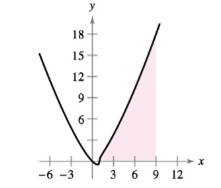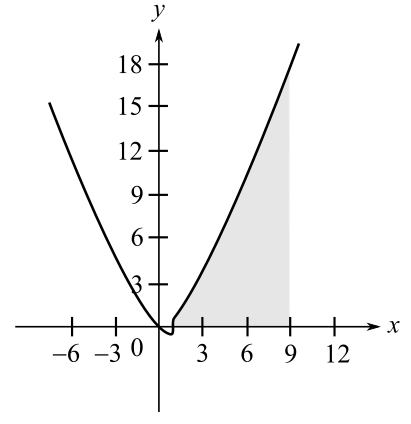Chapter 4, Problem 73RE

Chapter
Section
Textbook Problem

Finding the Area of a Region In Exercises 73 and 74, find the area of the region. Use a graphing utility to verify your result ∫ 1 9 x x − 1 3   d xTo determine

To calculate: The value of the integral 19xx13dx that gives the area of the shaded region:Explanation

Given:

The integral 19xx13dx.

Formula Used:

The area below the curve y=f(x) and above the x-axis when x lies in the interval [a,b] is given by:

A=abf(x)dx

Calculation:

Define the following variable:

x1=udx=du

When x=1,u=0 and when x=9,u=8.

Use this to rewrite the integral as:

19xx13dx=08(u+1)u13du

Now, thus can be evaluated as:

08(u+1)

Still sussing out bartleby?

Check out a sample textbook solution.

See a sample solution

The Solution to Your Study Problems

Bartleby provides explanations to thousands of textbook problems written by our experts, many with advanced degrees!

Get Started

x3a+b3

Applied Calculus for the Managerial, Life, and Social Sciences: A Brief Approach

In problems 31-38, write the equation of each line described. 34. Through and

Mathematical Applications for the Management, Life, and Social Sciences

Determine the size of 2, 3, and 4.

Mathematics For Machine Technology